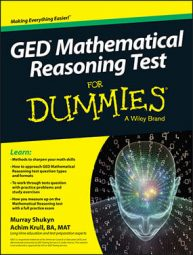##### GED Mathematical Reasoning Test For DummiesMany of the questions on the GED Mathematical Reasoning test are word problems. They describe a real-world problem that requires math to solve. You need to read and understand the problem before you can answer it. Here’s a good way to approach word problems:

The answer choices often provide valuable clues to solving the problem, such as the unit (miles, minutes, whatever) the answer needs to be in.

2. Jot down the values you know and assign a variable, such as x, to the value you don’t know.

3. Draw a picture if you think it will help.

This isn’t helpful for all questions, but it is for many of them.

4. Write the variable you assigned to the unknown value you need to find followed by the equal sign; for example, a = .

5. After the equal sign, write the equation as if it were a sentence using the values given.

For example: Henry had \$5 more in his pocket than Joe had. How much money did Henry have if Joe had \$12? If you let a stand for the amount of money that Henry had, then a = 12 + 5.

6. Solve the equation.

7. Select the answer choice that matches the answer you arrived at.

If you don’t know the answer, guess. Use what you know from reading the question and trying to solve the problem to try to eliminate one or more answers that appear to be obviously wrong, and then select from the remaining answer choices. You’re not penalized for guessing.